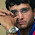VHDL coding tips and tricks: A VHDL Function for finding SQUARE ROOT

## Thursday, March 18, 2010

### A VHDL Function for finding SQUARE ROOT

I have written a function for finding the square root of a unsigned number in VHDL.The function is based on "Non-Restoring Square Root algorithm".You can learn more about the algorithm from this paper.The function takes one unsigned number,which is 32 bit in size and returns the square root,which is also of unsigned type with 15 bit size.The block diagram of the algorithm is given below:

Here D is the unsigned input number.R is the remainder of the operation for non-perfect squares. Q contains the square root of 'D'.
The function is given below:

library IEEE;
use IEEE.std_logic_1164.all;
use ieee.numeric_std.all;    -- for UNSIGNED

function  sqrt  ( d : UNSIGNED ) return UNSIGNED is
variable a : unsigned(31 downto 0):=d;  --original input.
variable q : unsigned(15 downto 0):=(others => '0');  --result.
variable left,right,r : unsigned(17 downto 0):=(others => '0');  --input to adder/sub.r-remainder.
variable i : integer:=0;

begin
for i in 0 to 15 loop
right(0):='1';
right(1):=r(17);
right(17 downto 2):=q;
left(1 downto 0):=a(31 downto 30);
left(17 downto 2):=r(15 downto 0);
a(31 downto 2):=a(29 downto 0);  --shifting by 2 bit.
if ( r(17) = '1') then
r := left + right;
else
r := left - right;
end if;
q(15 downto 1) := q(14 downto 0);
q(0) := not r(17);
end loop;
return q;

end sqrt;

The function can be used as follows in your main module:

--An example of how to use the function.
signal a : unsigned(31 downto 0) :="00000000000000000000000000110010";   --50
signal b : unsigned(15 downto 0) :=(others => '0');
b <= sqrt ( a );  --function is "called" here.
--b will contain the value "00000111" ( equals to 7) once the operation is done.

For using the function you have to copy the code snippet between the green lines and put it in a package.If you don't know about how to include functions in packages then you can learn it here.
Note :- This function is synthesizable.

#### 11 comments:

1.Hye GURU!!! I'm a beginner in VHDL. i have a coding for square root operation. i key in data for D and the answer appears in square root. so half of my job i done.. but the prob is, i need to interface with a keypad (to key in input for D) and display at LCD (output). somethng like a calculator.. can u help me with the VHDL code.. i'm using a 3x3 keypad and spartan 3 starter kit LCD. Help me plizz!!! thnks =)

2.I'm really confused about your construct ...

for i in 0 to 15 loop
[...]
q(15 downto 1) := q(14 downto 0);
q(0) := not r(17);
end loop;

As I know you would get 16 times
q(15 downto 1) := q(14 downto 0);
q(0) := not r(17);
as it is synthesized as parallel copies ...

Don't get, how you can iteratively shift your Q without clock and just with a for loop ...

I would be very interested about helping me out with this ...

Best regards
Thomas

3.@thomas : Good Question.
As I am using a function for the square root operation,there is no clock involved.It is a purely a combinational circuit.When synthesised Xilinx ISE uses LUT's(Look up tables) and some MUX'x for implementing it in hardware.
If you try synthesising it yourself you can see that a group of LUT's and MUX'x are connected in a cascaded fashion.This means that the logic written inside 'for loop' is implemented 15 times to realize the logic without clock.As you can see that this uses so much resources,but using functions is an easy way to write codes.
If you are concerned about the over use of logic gates, use a clock to implement the logic.This may reduce the logic gate usage by approximately 15.
(Note :- Shifting is not done in parallel here.)

4.while execution it is giving error . that is expecting entity or architecture near the function

with regards ,
nagaraja.v

5.the error came from function.... so, plz check it and reply to me.........

6.This code is working. But if you still need help regarding anything I can help for a fee.

7.I'm trying to use your code in my application with using Altera DE2. In my system fpga gets adc out from GPIO pins. Then fpga have to calculate sqrt. I have been using fixed_pkg.vhdl. When i send any fixed point number to your function after conversion to fixed point to unsigned, it works. But when i send the adc value, it doesn't. And says "Error: In lpm_divide megafunction, LPM_WIDTHN must be less than or equals to 64". Could you please help or advise something to me?

8.Hi, i have the same problem with you. If you find a solution, please write for me. I need help.

1.to bedri please

9.Could this code be easily adapted to deal with 32 bit fixed point numbers?

10.Does your code gives the output for non perfect square????? ..i.e; sqrt (50)= 7.07